# Reproducing a transport instability in convection-diffusion equation

Drawing from Larson-Bengzon FEM book, I wanted to experiment with transport instabilities. It looks there might be an instability in my ocean-ice model but before being able to address that, I wanted to wrap my head around the 1D simplest example one could find. And that is the Convection-Diffusion Equation:

(1)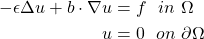The first term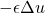is responsible of the smearing of the velocity field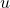proportionally to, and is thus called the diffusion term. Intuitively, it controls how much the neighbors of a given pointare influenced by the behavior of; how much velocities (or temperatures: think of whatever you want!) diffuse in the domain.

The second term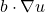controls how much the velocitiesare transported in the direction of the vector field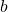, and is thus called the convective term. A requirement for this problem to be well-posed is that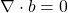— otherwise it would mean that we allow velocities to vanish or to come into existence.

The instability is particularly likely to happen close to a boundary where a Dirichlet boundary condition is enforced. The problem is not localized only close to the boundary though, as fluctuations can travel throughout the whole domain and lead the whole solution astray.

## Transport instability in 1D

The simplest convection-diffusion equation in 1D has the following form:

(2)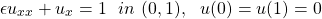whose solution, for smallis approximately just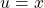. This goes well with the boundary condition at 0, but not with the condition at 1, where the solution needs to go down to 0 quite abruptly to satisfy the boundary condition.

It’s easy to simulate the scenario with FEniCS and get this result (with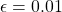and the unit interval divided in 10 nodes):in which we can infer two different trends: one with odd points and one with even points! In fact, if we discretize the 1D equation with finite elements we obtain:

(3)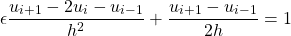aha! From this we see that, ifis not of the same order of magnitude of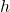(i.e. if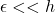), then the first factor becomes negligible. The problem then is that the second term contains only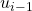and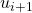. but not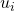. This will make it such that each node only talks to its second closest neighbor, explaining the behavior we saw in the plot before. It’s like even nodes make one solution and odd nodes a separate solution!

If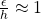, the solution that comes out is quite different:As we expected: a linear solution rapidly decaying towards the right. This is because in the above plot we had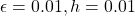, i.e. unit interval divided in 100 nodes.
Also notice how the problem does not pop up if the boundary conditions agree with the ideal solution (i.e. if the BC on the right is 1 instead of 0).

### Solving the transport instability with a stabilization coefficient

The easiest dynamic way to fix the issue is to introduce an artificial component toto make sure that the first term of the transport equation is never neglected, regardless of the relationship between mesh sizeand. This is a stabilization parameter:

(4)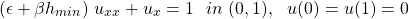where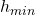is the mesh smallest diameter. There is no single correct value for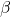: it quite depends on the other values (although it must be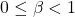). Anyway, a good starting point is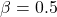, which can then be tweaked according to results. This way feels a bit hacky though: “if we can’t solve it for, let’s bump it up a bit” is pretty much the idea behind it.

With this formulation it’s also possible to derive what mesh size is needed to actually use a particular value for. For example, if we’d like the second derivative term to have a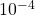coefficient, then we need a mesh size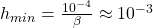, achieved with a 300×300 mesh, for example (which you can find out with
m=300; mesh=fenics.UnitSquareMesh(m,m); mesh.hmin()
). A uniformly fine mesh might not be needed though: it is often enough to have a coarse mesh in points where not much is happening, and very fine at problematic regions (such as boundaries, for this example).

### Code — Convection-diffusion equation 1D

from fenics import *
import matplotlib.pyplot as plt

mesh = UnitIntervalMesh(100)
V = FunctionSpace(mesh, 'P', 1)

bcu = [
DirichletBC(V, Constant(0), 'near(x, 0)'),
DirichletBC(V, Constant(0), 'near(x, 1)'),
]
u = TrialFunction(V)
v = TestFunction(V)
u_ = Function(V)
f = Constant(1)
epsilon = Constant(0.01)
beta = Constant(0.5)
hmin = mesh.hmin()

a = (epsilon+beta*hmin)*dot(u.dx(0), v.dx(0))*dx + u.dx(0)*v*dx
L = v*dx

solve(a == L, u_, bcs=bcu)

print("||u|| = %s, ||u||_8 = %s" % ( \
round(norm(u_, 'L2'), 2), round(norm(u_.vector(), 'linf'), 3)
))

fig2 = plt.scatter(mesh.coordinates(), u_.compute_vertex_values())
plt.savefig('velxy.png', dpi = 300)
plt.close()

#plot(mesh)
#plt.show()